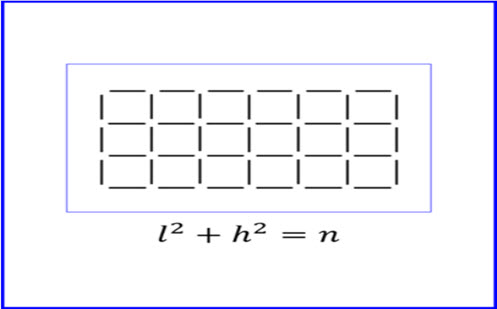Go to website

# Rectangle formula

Students first need to identify the meaning of the variables in the prompt in relation to the diagram. Students then pose questions and with the guidance of the teacher explore their questions. The resource includes the prompt, teacher notes, guided questions and examples of student solutions. There are also extension suggestions.

Year level(s) Year 9
Audience Teacher
Teaching strategies and pedagogical approaches Collaborative learning, Differentiated teaching, Explicit teaching, Feedback, Mathematics investigation
Keywords rectangular array, square number, formula, substitution

## Curriculum alignment

Curriculum connections Critical and creative thinking, Numeracy
Strand and focus Number, Apply understanding, Build understanding
Topics Algebraic expressions
AC: Mathematics (V9.0) content descriptions
AC9M9A04
Identify and graph quadratic functions, solve quadratic equations graphically and numerically, and solve monic quadratic equations with integer roots algebraically, using graphing software and digital tools as appropriate

Numeracy progression Number patterns and algebraic thinking (P9)